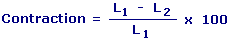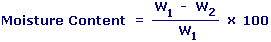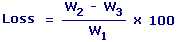Heat Effect On Ceramic Materials Enter value and click on calculate. Result will be displayed.

Calculate Contraction
Calculate Moisture Content
Calculate Loss On Ignition

 Web www.calculatoredge.com

Calculate ContractionL1 = Seperation of Test Marks on Sample in Initial State L2 = Seperation of Test Marks on Sample in Final State
 Enter your values: Initial Value (L1): Final Value (L2): Result: Contraction: %

Calculate Moisture ContentW1 = Wet Weight W2 = Weight of Sample after drying at 110°C
 Enter your values: Wet Weight (W1): Weight After Drying (W2): Result: Moisture Content: %

Calculate Loss On IgnitionW1 = Wet Weight W2 = Dried Weight W3 = Weight of Sample after Heating to about 1000°C
 Enter your values: Wet Weight (W1): Dried Weight (W2): Weight After Heating (W3): Result: Loss on Ignition: %LINKSDISCLAIMERCONTACT US